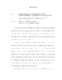## APPROXIMATION ALGORITHMS FOR POINT PATTERN MATCHING AND SEARCHI NG

 dc.contributor.advisor Mount, David M en_US dc.contributor.author Cho, Minkyoung en_US dc.contributor.department Computer Science en_US dc.contributor.publisher Digital Repository at the University of Maryland en_US dc.contributor.publisher University of Maryland (College Park, Md.) en_US dc.date.accessioned 2010-10-07T06:09:54Z dc.date.available 2010-10-07T06:09:54Z dc.date.issued 2010 en_US dc.description.abstract Point pattern matching is a fundamental problem in computational geometry. For given a reference set and pattern set, the problem is to find a geometric transformation applied to the pattern set that minimizes some given distance measure with respect to the reference set. This problem has been heavily researched under various distance measures and error models. Point set similarity searching is variation of this problem in which a large database of point sets is given, and the task is to preprocess this database into a data structure so that, given a query point set, it is possible to rapidly find the nearest point set among elements of the database. Here, the term nearest is understood in above sense of pattern matching, where the elements of the database may be transformed to match the given query set. The approach presented here is to compute a low distortion embedding of the pattern matching problem into an (ideally) low dimensional metric space and then apply any standard algorithm for nearest neighbor searching over this metric space. This main focus of this dissertation is on two problems in the area of point pattern matching and searching algorithms: (i) improving the accuracy of alignment-based point pattern matching and (ii) computing low-distortion embeddings of point sets into vector spaces. For the first problem, new methods are presented for matching point sets based on alignments of small subsets of points. It is shown that these methods lead to better approximation bounds for alignment-based planar point pattern matching algorithms under the Hausdorff distance. Furthermore, it is shown that these approximation bounds are nearly the best achievable by alignment-based methods. For the second problem, results are presented for two different distance measures. First, point pattern similarity search under translation for point sets in multidimensional integer space is considered, where the distance function is the symmetric difference. A randomized embedding into real space under the L1 metric is given. The algorithm achieves an expected distortion of O(log2 n). Second, an algorithm is given for embedding Rd under the Earth Mover's Distance (EMD) into multidimensional integer space under the symmetric difference distance. This embedding achieves a distortion of O(log D), where D is the diameter of the point set. Combining this with the above result implies that point pattern similarity search with translation under the EMD can be embedded in to real space in the L1 metric with an expected distortion of O(log2 n log D). en_US dc.identifier.uri http://hdl.handle.net/1903/10944 dc.subject.pqcontrolled Computer Science en_US dc.subject.pquncontrolled computational geometry en_US dc.subject.pquncontrolled geometric retrieval en_US dc.subject.pquncontrolled image registration en_US dc.subject.pquncontrolled point pattern matching en_US dc.title APPROXIMATION ALGORITHMS FOR POINT PATTERN MATCHING AND SEARCHI NG en_US dc.type Dissertation en_US
##### Original bundle
Now showing 1 - 1 of 1